# Solution assignment 03 Fractional functions and graphs

### Assignment 3

Given the function: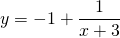Find:
the vertical asymptote;
the horizontal asymptote;
the intersection point with the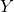-as if it exists;
the intersection point with the-as if it exists.

Based on these results sketch the result in the figure.

### Solution

The vertical asymptote is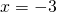. We find the horizontal asymptote whenor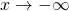and that is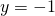, because the second term approaches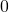and thus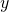approaches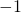. The intersection point with the-axis is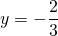. This result can be obtained by substituting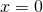in the function. The intersection point with the-as is obtained by substituting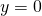and solving the following equation:The solution is: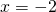See the graph in the following figure: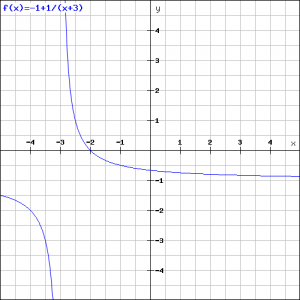0